Next: Linear Operators Up: Operators Previous: Operators and Quantum Mechanics

### Basic Properties of Operators

Most of the properties of operators are obvious, but they are summarized below for completeness.

• The sum and difference of two operators
and
are given by
=
(33)
=(34)

• The product of two operators is defined by
(35)

• Two operators are equal if
(36)

for all functions f.

• The identity operator
does nothing (or multiplies by 1)
(37)

A common mathematical trick is to write this operator as a sum over a complete set of states (more on this later).
(38)

• The associative law holds for operators
(39)

• The commutative law does not generally hold for operators. In general,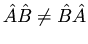. It is convenient to define the quantity
(40)

which is called the commutator ofand. Note that the order matters, so that
. Ifandhappen to commute, then
.

• The n-th power of an operator
is defined as nsuccessive applications of the operator, e.g.
(41)

• The exponential of an operator
is defined via the power series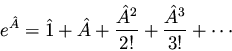(42)Next: Linear Operators Up: Operators Previous: Operators and Quantum Mechanics